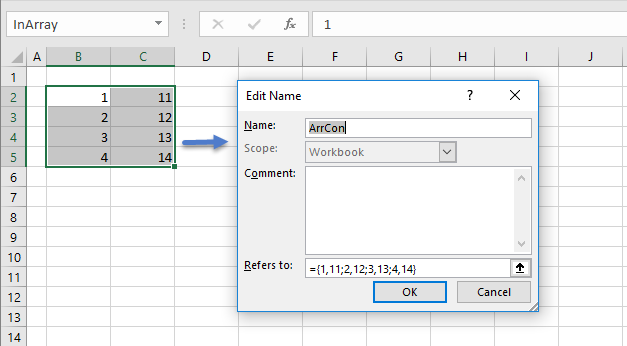# Write a worksheet array to named array constant

### [This is a project seed module]

This module provides a Quick HowTo:

• use VBA, to write an Excel numeric array to a named array constant (figure 1)
• sample VBA code is supplied (code 1)

## 0. PreliminaryFig 1: Numeric array to named array constant - the InArray range (see the Name Box) is assigned to the ArrCon defined name

## 1. The VBA code

Code 1: Macro xlArr2ArrC write the Excel range inArray to a named constant ArrCon
Sub xlArr2ArrC()
' Write an Excel numeric array - named inArray
' to a named array constant - with name ArrCon
' Details in Name Manager, Name: ArrCon; RefersTo: ={...}

Dim NoRows As Integer, NoCols As Integer
Dim ArrCon As String                ' Array constant
Dim Sep As String                   ' Element separator & row terminator
Dim i As Integer, j As Integer      ' Loop counters
Dim InArr As Range

Set InArr = [inArray]           ' a Range in Excel
ArrCon = "={"                   ' Initialize the array string
NoRows = InArr.Rows.Count
NoCols = InArr.Columns.Count

For i = 1 To NoRows
For j = 1 To NoCols
If j < NoCols Then
Sep = ","
ElseIf i < NoRows Then
Sep = ";"
Else
Sep = ""
End If
ArrCon = ArrCon & InArr(i, j) & Sep
Next j
Next i

ArrCon = ArrCon & "}"                     ' Terminate the array string